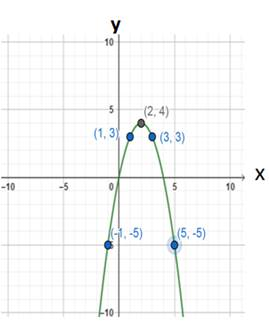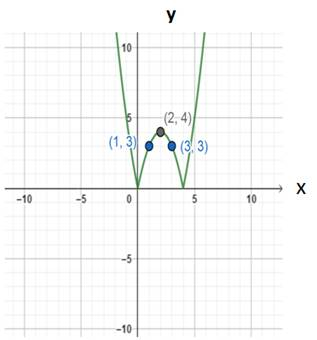# To sketch the graph of function### Precalculus: Mathematics for Calcu...

6th Edition
Stewart + 5 others
Publisher: Cengage Learning
ISBN: 9780840068071### Precalculus: Mathematics for Calcu...

6th Edition
Stewart + 5 others
Publisher: Cengage Learning
ISBN: 9780840068071

#### Solutions

Chapter 2.5, Problem 87E

a.

To determine

## To sketch the graph of function

Expert Solution

### Explanation of Solution

Given information : The function is f(x)=4xx2

Graph : This function by plotting points

Make a table of values of the function for several values of x

 x -1 0 1 2 3 4 5 4x−x2 -5 0 3 4 3 0 -5Interpretation : The above graph of function f(x)=4xx2

From the above graph the points are

(1,3)(3,3)(1,5)(5,5)

To determine

Expert Solution

### Explanation of Solution

Given information : The function is g(x)=|4xx2|

Graph : This function by plotting points

Make a table of values of the function for several values of x

 x 2 1 3 |4x−x2| 4 3 3Interpretation : The above graph of function g(x)=|4xx2| .

From the above graph the points are

(1,3)(3,3)

### Have a homework question?

Subscribe to bartleby learn! Ask subject matter experts 30 homework questions each month. Plus, you’ll have access to millions of step-by-step textbook answers!